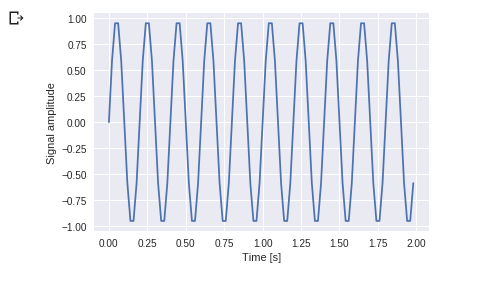Home » Blog » Discrete Fourier Transform – scipy.fftpack

# Discrete Fourier Transform – scipy.fftpack

## scipy.fftpack

• DFT is a mathematical technique which is used in converting spatial data into frequency data.
• FFT (Fast Fourier Transformation) is an algorithm for computing DFT
• FFT is applied to a multidimensional array.
• Frequency defines the number of signal or wavelength in particular time period.

Example: Take a wave and show using Matplotlib library. we take simple periodic function example of sin(20 × 2πt)

```%matplotlib inline
from matplotlib import pyplot as plt
import numpy as np

#Frequency in terms of Hertz
fre  = 5
#Sample rate
fre_samp = 50
t = np.linspace(0, 2, 2 * fre_samp, endpoint = False )
a = np.sin(fre  * 2 * np.pi * t)
figure, axis = plt.subplots()
axis.plot(t, a)
axis.set_xlabel ('Time (s)')
axis.set_ylabel ('Signal amplitude')
plt.show()```

Output :You can see this. Frequency is 5 Hz and its signal repeats in 1/5 seconds – it’s call as a particular time period.

Now let us use this sinusoid wave with the help of DFT application.

```from scipy import fftpack

A = fftpack.fft(a)
frequency = fftpack.fftfreq(len(a)) * fre_samp
figure, axis = plt.subplots()

axis.stem(frequency, np.abs(A))
axis.set_xlabel('Frequency in Hz')
axis.set_ylabel('Frequency Spectrum Magnitude')
axis.set_xlim(-fre_samp / 2, fre_samp/ 2)
axis.set_ylim(-5, 110)
plt.show()```

Output:• You can clearly see that output is a one-dimensional array.
• Input containing complex values are zero except two points.
• In DFT example we visualize the magnitude of the signal.